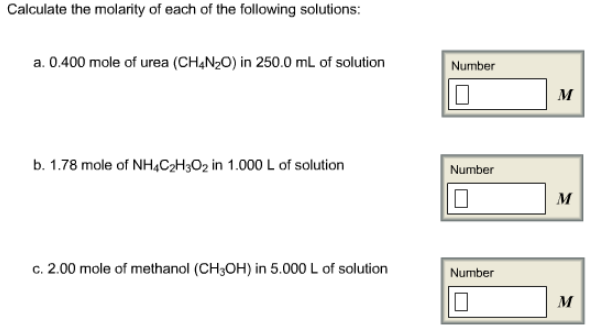Chemistry Molarity Solution: Calculate the molarity of each of the following so...

🤓 Based on our data, we think this question is relevant for Professor Webb's class at ISU.

# Solution: Calculate the molarity of each of the following solutions: a. 0.400 mole of urea (CH4N2O) in 250.0 mL of solution b. 1.78 mole of NH4C2H3O2 in 1.000 L of solution c. 2.00 mole of methanol (CH 3OH) in 5.000 L of solution

Problem

Calculate the molarity of each of the following solutions:

a. 0.400 mole of urea (CH4N2O) in 250.0 mL of solution

b. 1.78 mole of NH4C2H3O2 in 1.000 L of solution

c. 2.00 mole of methanol (CH 3OH) in 5.000 L of solutionView Complete Written Solution